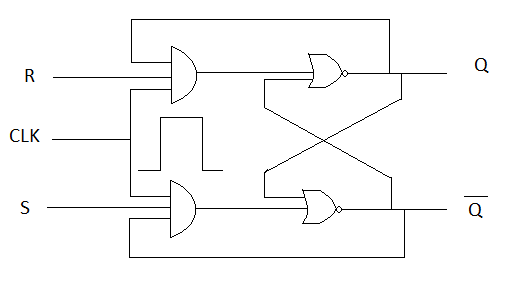## Latest:

latest

### Flip-Flop is an element that is built by using logical gates. It is a memory element that can save a single bit (0 or 1). In a computer mem...

Flip-Flop is an element that is built by using logical gates. It is a memory element that can save a single bit (0 or 1). In a computer memory, the main element is a flip-flop.

## Flip-Flop

In digital electronics, which circuit works on an active condition and an inactive condition that is called a multivibrator. We can find many types of multivibrators. But from these which multivibrators 2 condition stays fixed, that is a flip-flop. In flip-flop, 2 conditions stay fixed. It can save one bit(0 or 1) permanently. In flip-flop, there are 2 outputs. If one is Q then another one is Q̄.

### Uses of Flip-Flop

1. As an element of memory in most cases
2. For making the counters

5 types of Flip-Flops are:

1. SR Flip-Flop
2. JR Flip-Flop
3. D Flip-Flop
4. T Flip-Flop
5. Master / Slave Flip-Flop

### SR Flip-Flop

It is the most simple Flip-Flop. It is a Set Reset Flip-Flop. Here if the process is making the output condition 1 or high then it is called Set. Again, if the process is making the output condition 0 or low then it is called Reset.

SR Flip-Flop has 3 types. they are-

1. NAND latch SR Flip-Flop
2. NOR latch SR Flip-Flop
3. Clocked SR Flip-Flop

#### NAND latch SR Flip-Flop

If two NAND gate connects together as the input of one NAND gate is connected with the output of another NAND gate then it is called NAND latch SR Flip-Flop.

#### NOR latch SR Flip-Flop

If two NOR gate connects together as an input of one NOR gate is connected with the output of another NOR gate then it is called NOR latch SR Flip-Flop.

*Clock pulse: It is a type of attendance of signal or voltage which will keep the same in some time and after that again it will be in other conditions at the same time.

#### Clocked SR Flip-Flop

In  Clocked SR Flip-Flop there will two inputs S, R, and a clock pulse CLK. It is also called CP. S(Set) and R(Reset) inputs are sent in Flip-Flop input by AND operation of AND gate.

In the figure, we are seeing Clocked SR Flip-Flop.
When CP = 1 then that time enters R and S Flip-Flop input and changes the output.The internal structure of Clocked flip flop

#### A truth table for Clocked S-R Flip Flop

 Inputs Outputs S R CLK Q 0 0 ↑ Unchanged 0 1 ↑ 0 1 0 ↑ 1 1 1 ↑ Uncertain

#### Block diagram of SR Flip Flop

Working procedure :

1. Set = 0 and Reset = 0 . It is the normal condition of S-R Flip Flop which will not play any role in its output.
2. Set = 0 and Reset = 1. In this case, if a clocked pulse is given then it will change output in Q=0 state. It will reset SR Flip Flop.
3. Set = 1 Reset = 0. If a clocked pulse is given, it will change the SR flip flop output condition into a Q=1 state. It will set the SR flip-flop.
4. Set = 1 Reset = 1.In this situation, after giving clocked pulse uncertain results will come. That means in this case output Q and Q̄ will be tried to be set at the same time Q=Q̄=1 which will not be considering the rule of Flip Flop. This condition is called a Race condition. For this disadvantage, this condition of SR flip flop is not used.

#### Disadvantages of SR Flip Flop

For S = 1 and R = 1 first system will be 0 or 1. But next, it will show an uncertain situation. That's why JK Flip Flop is used.

### JK Flip-Flop

JK Flip-Flop is the updated version of SR Flip-Flop. In SR Flip-Flop output for input S= R=1 problem solving it has made by little changing.

Truth table: JK Flip-Flop

 Inputs Outputs S R CLK Q₀ 0 0 Q₀ Unchanged 0 1 Always 0 1 0 Always 1 1 1 Q̄₀ (Toggle)

Block diagram of JK Flip-Flop

#### Working procedure:

As like SR Flip Flop. Only when J =1 and K = 1 input and if the clock pulse is given in CLK then the output will toggle in every time. That means before giving input the output will Q and after inputting the present output will Q̄₀.

### D Flip-Flop

By changing a little bit in Clocked SR Flip-Flop D Flip-Flop has been built. Adding an inverter into SR Flip Flop has been made. That's why it's one input is always different from another. It has only a single input.

Mainly it is a Clocked SR Flip Flop in which S=D and R = D.
In the figure, D Flip-Flop is showed below.

#### D Flip-Flop truth table

 D CLK Q 0 ↑ 0 1 ↑ 1

In D Flip-Flop output does not depend on previous output. Output and input are equal always. Output = input that's why it is called Transparent.
Normal equation : Q(t+1)= D.

### Clocked T Flip-Flop

Clocked T Flip-Flop has been created by little changing the JK Flip-Flop. It is called the single input JK Flip-Flop. For JK Flip-Flop J = K =T. For Clocked T Flip-Flop T =1 then its output will always toggle.

In Clocked T Flip-Flop if T =1 then Q₀ = Q̄.

#### A truth table for Clocked T Flip-Flop

 T Q 0 Q 1 Q̄₀